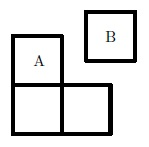Tiling

Probability Level 5

A $2 \times 2$ square is cut into two pieces, $A,$ which has area 3, and $B,$ which is a square of area $1,$ as shown in the figure below.A $2 \times 8$ grid is to be tiled with various pieces having shape $A$ or $B.$ Pieces of shape $A$ can be rotated by $0, \frac{\pi}{2}, \pi, \mbox{ or } \frac{3\pi}{2}$ before being placed. Subject to the condition that three pieces of shape $B$ cannot occur on the grid in such a way that they could be replaced by a piece of shape $A$, how many different tilings are there of the grid?

×